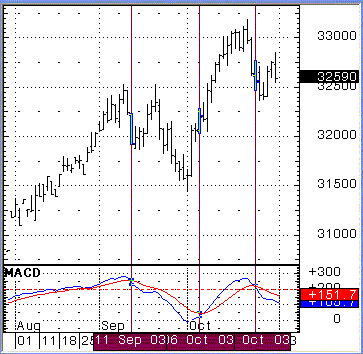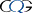This is one of the most commonly used indicators in the chartist's arsenal. Developed by Gerald Appel, the indicator is derived by plotting the difference between two exponential moving averages, with the default parameters of 12 and 26 periods. A second line, the trigger line, is calculated by using a shorter period moving average of the first line, which uses 9 periods by default.

The first line, the MACD is a simple calculation:

MACD = EMA (12) - EMA (26)

The second line is the exponential moving average of the first line

MACDA (n) = MACD (n-1) + [k * (MACD (n) - MACD (n-1)

• where n is the current period
• k is a smoothing constant (refer to section on Exponential Moving Average)
• and 9 is the number of MACD data points used to calculate the MACDA© Copyright 2003 CQG, Inc. All rights reserved worldwide

### How to use it

The MACD can be used quite simply when employing a crossover method. A trading signal is generated when the fast line crosses the slow line, in the direction of the cross. In the above example the blue line is the fast MACD line and the red line is the slower MACDA line. A buy signal is generated when the blue line crosses above the red line and a sell signal is generated when the blue line crosses below the red line.

In the above example, the first crossover on the chart in September generated a sell signal, which was countered by a buy signal in Oct and another sell signal later in October. Each signal can be used as a trigger to take a new position, not necessarily as a stop or an exit for the previous signal.

Divergence means moving apart, while convergence means moving closer together. In this case we are referring to whether the two indicator lines are converging or diverging. Convergence of the indicators indicates trend change or consolidation of the current, recent trend. Divergence is associated with a developing trend, as the lines move further apart they indicate an accelerating market move, whereas when the lines are moving closer together they indicate the trend slowing.

The MACD is a boundless indicator so it's very dangerous to try and apply overbought/oversold characteristics to the market using this indicator, as it can just keep going. Therefore it's a great trend following indicator while at first, resembling an oscillator type indicator. We can use the zero line though and when the indicator crosses above or below the zero line we can take that as a possible change in longer term trend. If the MACD crosses above the zero line it indicates a bull market trend and below a bear market trend. In the above example all the activity is above the zero line so we're clearly in a bull market.

Despite the fact that it is a boundless indicator the market will tend to set up its own parameters. This is one of the benefits of watching the MACD rather then the two underlying moving averages that go make it up. Peaks in the indicator tend to happen at around the same level and therefore as the indicator approaches an old peak we can read that as potential for a change in the short term trend, as it has probably over-extended itself, relative to the longer term.

We can see in the above example that the blue line peaked just below +300 in early September, which established a reference high for the indicator. So when we see the indicator approach that level again in October, this time with price 50 points higher, we can look for associated signals of deterioration to issue a sell signal. That came in two ways, first by convergence of the two indicators and then by a cross of the two indicators. Nevertheless due to the absolute location of the indicator, well above the zero line, we can view this as a correction to the underlying bull market trend, rather than a complete change in that underlying trend.

Disclaimer
© The MacLean Group Pty Ltd ACN 096 967 038. All rights reserved 2003. This article has been prepared by The MacLean Group and licensed to ASX. The views are those of the author and not of ASX. This material is educational and it is not intended to constitute financial advice.